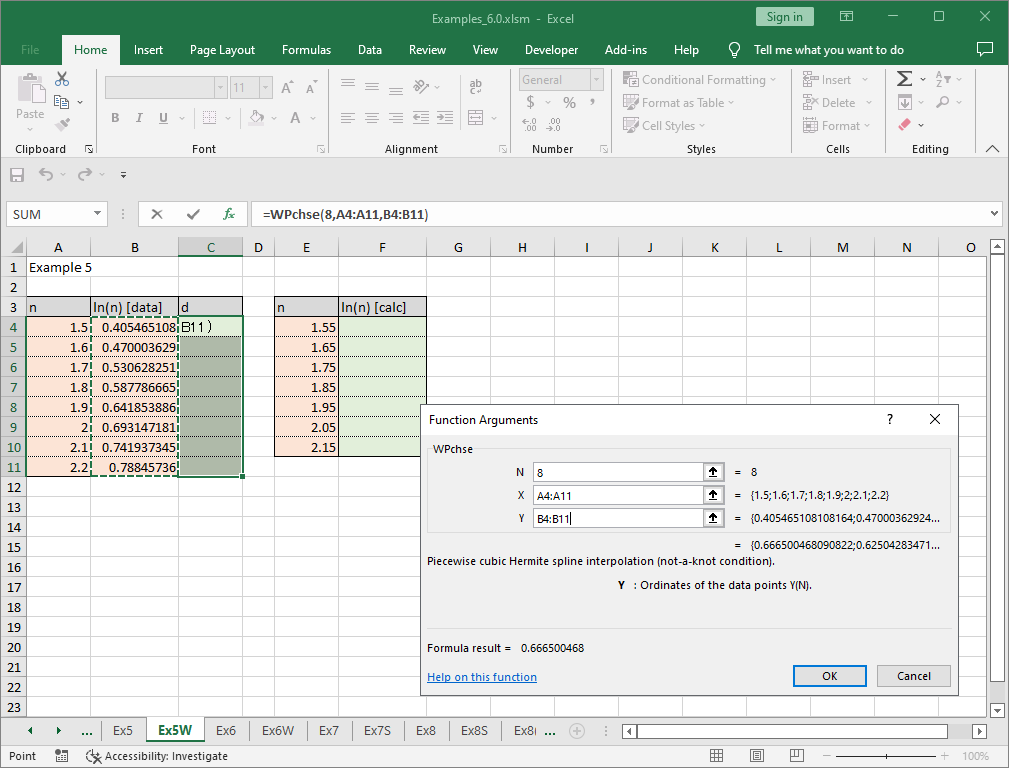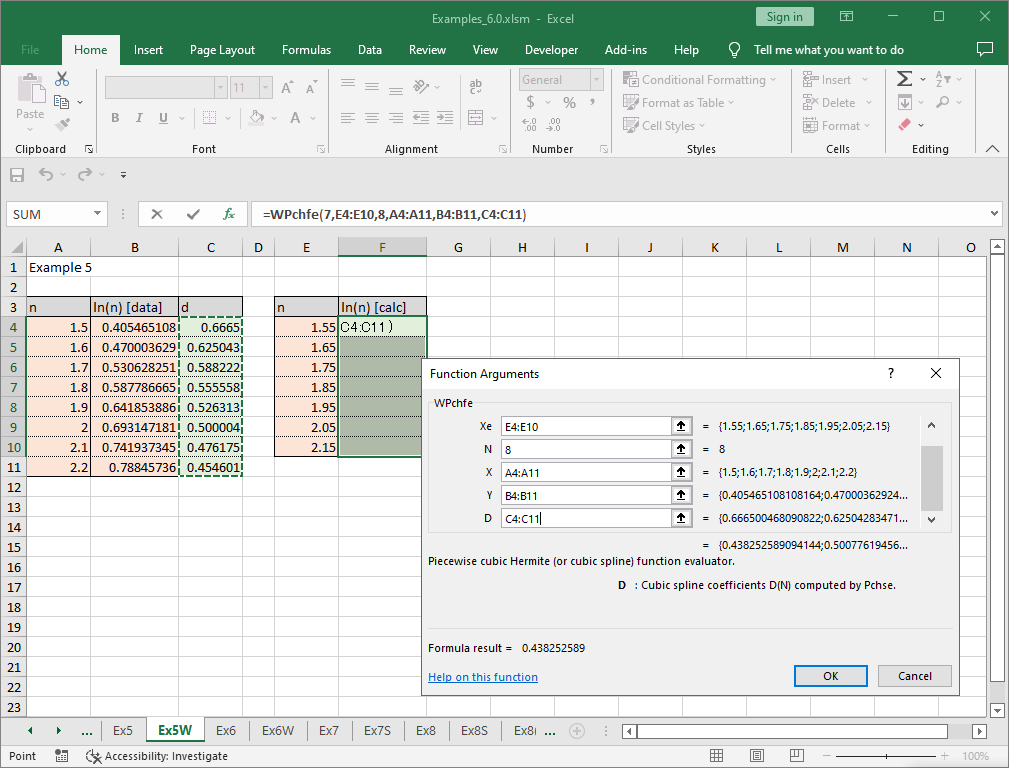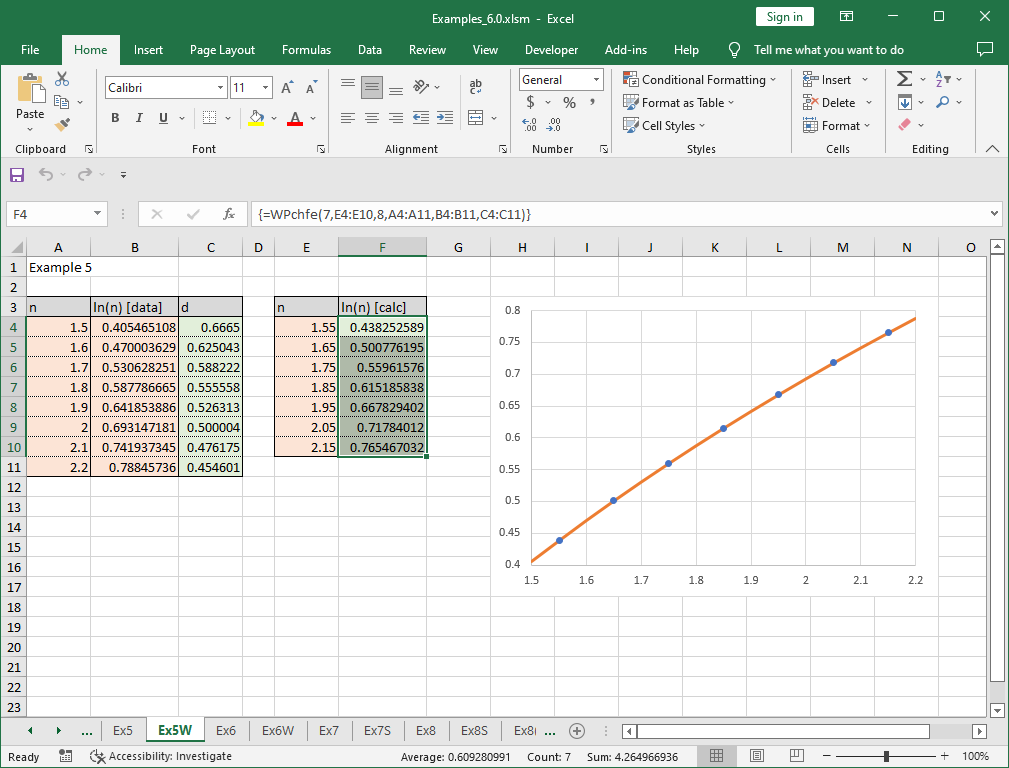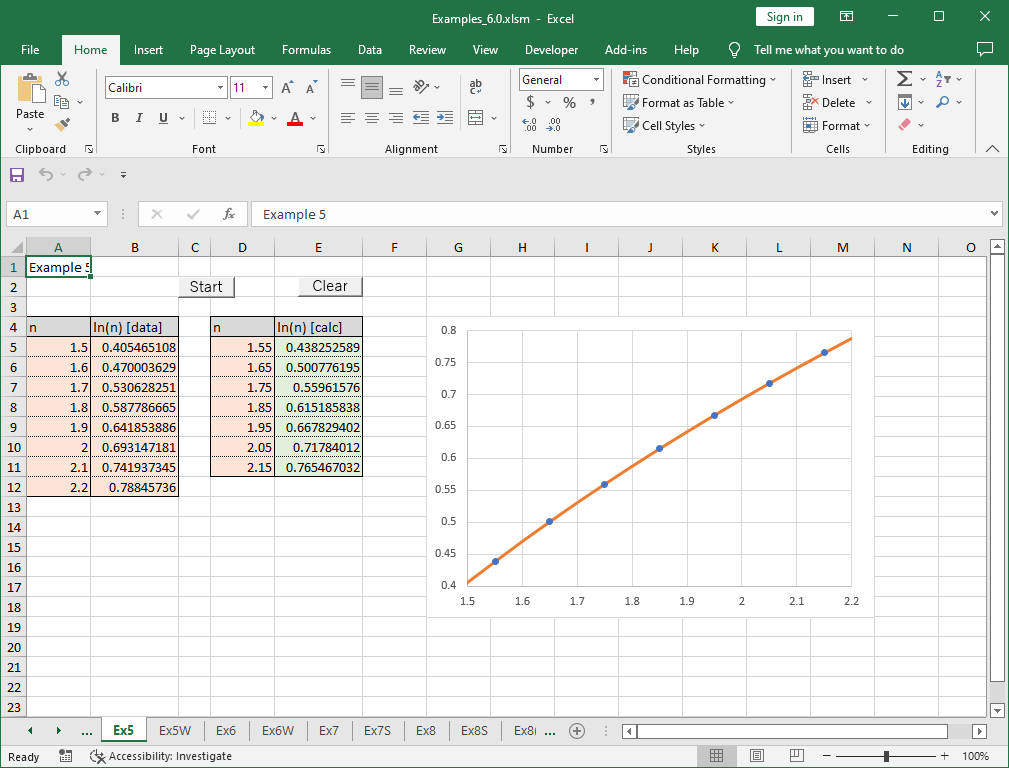# 5. Interpolation

Note – The example worksheets are available for download. See 1. How to obtain and use example worksheets.

Examples of computing interpolation by cubic spline function are shown below.

### Example: Logarithm table

Although it is no longer used these days, logarithm tables and other numerical tables were used in past days.

The table below is an excerpt from the table of natural logarithms.

n ln(n)
1.5 0.405465108108164
1.6 0.470003629245736
1.7 0.53062825106217
1.8 0.587786664902119
1.9 0.641853886172395
2.0 0.693147180559945
2.1 0.741937344729377
2.2 0.78845736036427
Suppose we want to find a value that is not listed in the numerical table, such as ln(1.85).

Two solving methods using worksheet function WPchse and WPchfe, and VBA subroutine Pchse and Pchfe are described below.

### Solving problem with worksheet function

Enter the data (xi, yi = ln(xi)) into the appropriate cells in the worksheet (left orange cells). xi must be in increasing order. Then select N (= number of data points) cells where the spline coefficients d are to be entered (left green cells) and enter woeksheet function WPchse.

The parameters required by WPchse are N, X and Y. N is the number of data points (= 8 for this example). X and Y are the cell range of data (xi, yi) to be interpolated.Press Ctrl + Shift + Enter, then spline coefficients are obtained.

Enter values of points where the interpolated values are to be computed in the other cells (for example, n = 1.55, 1.65, …, 2.15) (right orange cells). Then compute the function values for these points by using WPchfe worksheet function (right green cells).

The parameters required by WPchfe are Ne, Xe, N, X, Y and D. Ne is the number of evaluation points (= 7 for this example). Xe is the cell range of the points to be evaluated. N, X and Y are the same data used by WPchse when computing the spline coefficients. D is the cell range of obtained spline coefficients.Press Ctrl + Shift + Enter, then the natural logarithm values for the points not tabulated are obtained. In this example, the graph is also created. The obtained values are shown by the circles and the table values are shown by the line.Although data points are equally spaced in this example, they may be unequally spaced. However, the interpolated values for the interval with large space may be less accurate than those for short intervals. The values for the arguments of outside the data points (extrapolated values) can be computed but may be less accurate.

### Solving problem with VBA program

The following is an example code using VBA library subroutine Pchse and Pchfe.

``````Sub Start()
Const NMax = 10
Dim N As Long, Ne As Long, I As Long, Info As Long
Dim X(NMax) As Double, Y(NMax) As Double, D(NMax) As Double
Dim Xe(NMax) As Double, Fe(NMax) As Double
'--- Input data
N = 8
For I = 0 To N - 1
X(I) = Cells(5 + I, 1)
Y(I) = Cells(5 + I, 2)
Next
'--- Compute coefficients of a cubic spline
Call Pchse(N, X(), Y(), D(), Info)
If Info <> 0 Then
MsgBox "** Error in Pchse **  Info = " + Str(Info)
Exit Sub
End If
'--- Compute interporated values
Ne = N - 1
For I = 0 To Ne - 1
Xe(I) = Cells(5 + I, 4)
Next
Call Pchfe(N, X(), Y(), D(), Ne, Xe(), Fe(), Info)
If Info <> 0 Then
MsgBox "** Error in Pchfe **  Info = " + Str(Info)
Exit Sub
End If
'--- Output result
For I = 0 To Ne - 1
Cells(5 + I, 5) = Fe(I)
Next
End Sub``````

After entering the data into the appropriate cells (orange cells), the following result was obtained by running the macro “Start”. Unlike the example using worksheet functions, you cannot obtain results by simply entering the data, and you need to run a VBA program.